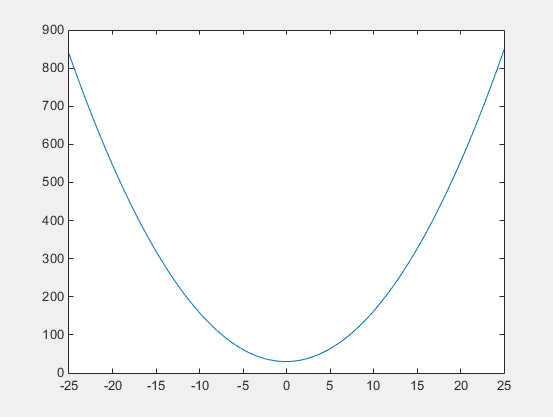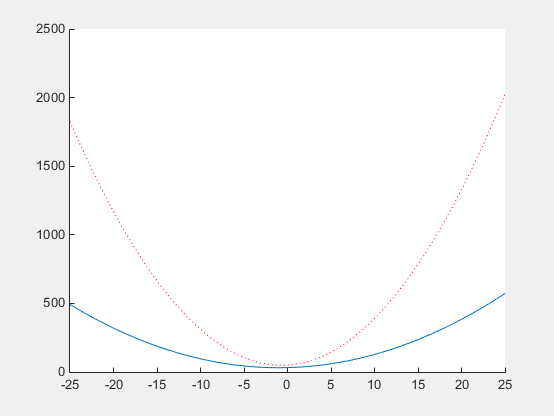## 嵌套函数

### 什么是嵌套函数？

```function parent disp('This is the parent function') nestedfx function nestedfx disp('This is the nested function') end end```

• 嵌套函数可以使用不是以输入参数形式显式传递的变量。

• 在父函数中，您可以为嵌套函数创建包含运行嵌套函数所必需的数据的句柄。

### 嵌套函数的要求

• 嵌套函数通常不需要 `end` 语句。但是，要在程序文件中嵌套任何函数，该文件中的所有函数都必须使用 `end` 语句。

• 不能在任何 MATLAB® 程序控制语句内定义嵌套函数，例如 `if/elseif/else``switch/case``for``while``try/catch`

• 必须按名称直接调用嵌套函数，而不使用 `feval` 或使用您使用 `@` 运算符创建的函数句柄（并不是 `str2func`）。

• 嵌套函数或包含嵌套函数的函数中的所有变量都必须显式定义。即，除非变量已存在于函数工作区中，否则无法调用函数或脚本向那些变量赋值。（有关详细信息，请参阅解决错误：尝试向静态工作区添加变量。。）

### 在父函数与嵌套函数之间共享变量

 ```function main1 x = 5; nestfun1 function nestfun1 x = x + 1; end end``` ```function main2 nestfun2 function nestfun2 x = 5; end x = x + 1; end```

```function main nestedfun1 nestedfun2 function nestedfun1 x = 1; end function nestedfun2 x = 2; end end```

```function parentfun x = 5; nestfun; function y = nestfun y = x + 1; end end```

```function parentfun x = 5; z = nestfun; function y = nestfun y = x + 1; end end```

### 使用句柄存储函数参数

• 输入参数

• 在嵌套函数内定义的变量

• 在父函数中定义的变量，还称为外部作用域变量。

```function p = makeParabola(a,b,c) p = @parabola; function y = parabola(x) y = a*x.^2 + b*x + c; end end```

`makeParabola` 函数返回 `parabola` 函数的句柄，该函数包含系数 `a``b``c` 的值。

```p = makeParabola(1.3,.2,30); X = 25; Y = p(X)```
```Y = 847.5000```

`fplot(p,[-25,25])````firstp = makeParabola(0.8,1.6,32); secondp = makeParabola(3,4,50); range = [-25,25]; figure hold on fplot(firstp,range) fplot(secondp,range,'r:') hold off```### 嵌套函数的可见性

• 紧邻的上一级。（在下面的代码中，函数 `A` 可以调用 `B``D`，但不能调用 `C``E`。）

• 在同一父函数内的同一级别嵌套的函数中。（函数 `B` 可以调用 `D`，而 `D` 可以调用 `B`。）

• 任何更低级别的函数中。（函数 `C` 可以调用 `B``D`，但不能调用 `E`。）

```function A(x, y) % Main function B(x,y) D(y) function B(x,y) % Nested in A C(x) D(y) function C(x) % Nested in B D(x) end end function D(x) % Nested in A E(x) function E(x) % Nested in D disp(x) end end end```